tech@7universum.com
+7 (499) 938-98-57

# Improvement of methods for calculating the maximum flow rate of mudflow floods and openings of bridges in the design of culverts on roads and railways

Усовершенствование методов расчёта максимальных расходов селевых паводков и отверствий мостов при проектировании водопропускных сооружений на автомобильных и железных дорогах
Цитировать:
Tulyaganov A.H., Mahkamov B.R. Improvement of methods for calculating the maximum flow rate of mudflow floods and openings of bridges in the design of culverts on roads and railways // Universum: технические науки : электрон. научн. журн. 2021. 5(86). URL: https://7universum.com/ru/tech/archive/item/11677 (дата обращения: 23.09.2021).
Прочитать статью:
DOI - 10.32743/UniTech.2021.86.5.11677

ABSTRACT

The article formulates the basic requirements for the design of small bridge crossings in the foothill mudflow channels. A new approach to hydrological calculations in determining their holes, based on morphometric dependence, is proposed. A method is given for calculating the maximum flow of mudflow and their parameters, subject to the law of “vertical zoning” characteristic of the foothill territories of the Republic of Uzbekistan.

АННОТАЦИЯ

В статье сформулированы основные требования к проектированию малых мостовых переходов в предгорных селевых руслах. Предлагается новый подход к гидрологическим расчетам при определении размеров мостового отверстия, основанный на морфометрической зависимости. Приведена методика расчета максимального стока селей и их параметров с учетом закона «вертикального зонирования», характерного для предгорных территорий Республики Узбекистан.

Key words: mudflow, maximum flow rate, selenium content coefficient; maximum 10-minute intensity, channel slope, flow rate

Ключевые слова: селевой поток, максимальный расход, коэффициент селевой активности; максимальная 10-минутная интенсивность, наклон канала, скорость потока

1. Introduction.

In practice, when designing road crossings through mudflow channels, designers usually take the characteristics of mudflows purely "water" flows, although they contain, in varying quantities, and a solid phase. As a result, on piedmont road structures this often leads to massive plugging of pipe holes and destruction of bridge supports, etc. (fig. 1).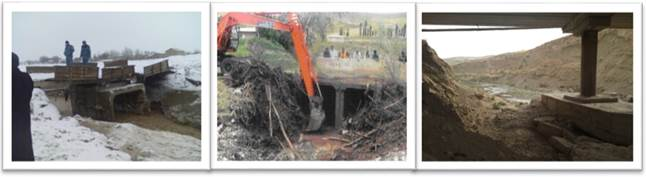Figure 1. Impact of mudflows on culverts

According to SM Fleishman , if the relative volume of the solid phase exceeds 10-15%, such a flood (which corresponds to the volume of watercourses in small catchments of the foothills of Uzbekistan ) refers to mudflows. From the point of view of Yu.M. Denisov , mudflows should be considered from a single point of view, as objects containing liquid and solid phases in different ratios. We have taken just such an approach in the development of this work. Note that the increase in the cases of mudflows in Uzbekistan [9,10,11] is caused by an increase in the amount, frequency and intensity of precipitation, as well as in connection with the intensity of the development of foothill territories; the issue of designing bridges and calculating the opening of bridge crossings acquires both scientific and practical interest.

2. Goals and objectives of the work.

The purpose and task of the work is to develop the definition of the opening of bridge crossings on small foothill mudflow channels. The object of research is the foothills of the Republic of Uzbekistan.

3. Main part (results and discussion).

The design of bridge crossings and calculations of the holes of road structures began in the 60s of the last century. These works are linked by the names of Russian scientists - O. V. Andreev, E. V. Boldakov, V.A. Bolshakov, A.A. Kurganovich and other authors [1,2,3,4]. However, in these and subsequent works [6,13,14,21], the calculations of the openings of bridge crossings in the case of mudflow channels were not considered.

As a result of visual surveys, culverts through mudflow channels and, on the basis of published works [21, 22], we formulated the following basic requirements for small bridge crossings, as well as the principles of hydrological and hydraulic calculations of their openings:

• bridge crossings over mudflow channels should be designed in areas where the longitudinal slope of the channel at the crossing section provides a transit passage of mudflows with the full flow depth;
• crossings of mudflow channels should be carried out normally to the dynamic axis of the flow at the design site;
• in other cases, either special measures should be provided for the arrest or passage of mudflows, or in the calculation formulas for determining the height of the hole, the increment of the mark of the bridge channel for the estimated period of operation of the structure should be taken into account;
• when the mudflow passage is constrained by the household flow width, a device is required to guide the structure from the entrance to the mudflow passage to the area where the flow channel is rigidly fixed by natural banks that do not have sharp breaks and turns.
• it is desirable to avoid intermediate supports when passing mudflows. If the implementation of intermediate supports is inevitable, one should strive to place them outside the dynamic axis of the flow - on the peripheral sections of the mudflow channel and give a zero width of the frontal edge of each intermediate support;
• it is not allowed to back up the mudflow in front of the entrance to the mudflow passage, since such backwater causes a blockage of mudflows in front of the structure.

The main design characteristics when calculating the openings of mudflow passages should be: design depth of mudflow –hcalc, width –Bcalc, design flowrate –Qcalc, and channel slope –J.

The initial material for the calculation are: the catchment area in front of the structure –F, the catchment height Z and the root-mean-square deviation of the height –σ. These characteristics are determined from a topographic map.

Let us describe the methodology for the approach of hydrological calculation of the openings of bridge crossings.

Let us consider in the case when the longitudinal slope of the watercourse ensures the pass of mudflows with the full depth hcalc through the mudflow check canal. In this case, the width of its Bcalc and hcalc according to S.M. Fleishman  shall be determined by the transfer of B and h from stable hydraulic gates, broken in the transit zone and reduced to the design capacity, while the flow depth shall be determined by the maximum (highest marks). According to the proposed option, the hydraulic parameters Bcalc and hcalc are recommended to be obtained from hydromorphological dependencies (such dependencies were used to calculate the maximum water discharge of rainfall floods ). For the sub mountain rivers of Uzbekistan, these dependencies shall have: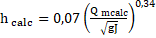,                                                                       (1)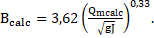(2)

where: Qm calc is the estimated maximum discharge of the mudflow flood; J is a channel slope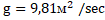;

When deriving these equations, we used the field data of G.V Vafin .

The slope of the watercourse is determined by the empirical formula:

J  = kJ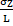=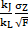,                                                                            (3)

where: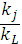- proportionality factor equal to 1.79.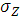is the standard deviation of the catchment height.

σz = 0.647ln (Z) + 0.061.                                                                       (4)

The maximum flow rate of a mudflow flood Qmс is calculated by the "indirect method", which is the multiplied water flow rate Qmв by the selenium density factor ψ:

Qmc = ψ Qmv.                                                                               (5)

In the literature, there are a number of dependencies for calculating rain maximums [1,7,13,15]. To calculate Qmv when determining the maximum discharge of rainfall flood, we adopted the formula we proposed, based on the "method of limiting intensity", derived for the conditions of small water collections from sub-mountains of Uzbekistan :

Qmах = 16,67 a10 kt F α φ.                                                                    (6)

Here ahour is the intensity of a shower with hourly duration, mm / min; kt is the transition factor from the intensity of 10 minutes duration to the intensity of the shower with estimated duration; F is the water collection area;

α is the flow factor; φ is the reduction factor.

Let us present the calculation of the parameters included in formula (6).

Maximum 10 minute rain intensity (a10). Maximum, 10-minute rain intensity, is determined by the expression:

a10 = m ί.                                                                                (7)

Here m is the transition factor from average to maximum rain intensity, taken equal to m = 4.

In the absence of pluviographs, the average rain intensity is found by the well-known formula: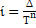,                                                                               ( 8 )

where Δ is the meteorological force of rain; T is the duration of the rain; n is the exponent.

The variable parameters in formula (8) ∆, n and T are determined by empirical formulas obtained for the territory of the foothills of Uzbekistan, governed by the law of “vertical zoning”, i.e. the change in these values ​​with increasing terrain height. Here are the calculation formulas and its correlations (R):

Meteorological rainfall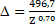, R = 0.66                                                                  (9)

where Z is the water collection height, m.

Exponent n and rain duration T

n = 0,52 ∆ 0,15 .              R = 0,57                                                    (10 )

ТД = Аr ∙ rb .               R = 0,91                                                       (11 )

In the formula (11) Аr is a unit of time measurement; b - parameter; in average, Ar = 15.1 and b = 2.01; r is the relative humidity of the air.

r = 0, 584  Z 0, 099..    R=0,82                                                       ( 12)

Transient factor of 10 minute intensity to intensity of calculated duration (kt). This parameter is closely related to the flow rate (v) and the length of the main watercourse (L). The value of the factor is determined by the formula:

k t =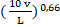,                                                                  (13)

The structure of the formula for calculating this speed is adopted in the form :

v = k J m km / min,                                                            (14)

where k is the factor associated with the channel roughness k = 0.22; J - watercourse slope; m - exponent, m = 0.201.

Runoff factor (α). According to our research, the runoff factor α is taken depending on the intensity of rain, so for the average intensity:

ίср< 0,5 mm/min α = 0,5 and ίср ˃ 0,5 mm/min α = 0,7.

Reduction factor (φ). The reduction factor (φ) is determined by the well-known formula:

φ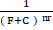=.                                                             (15)

where C is the correction factor to the area F, less sharp decrease in the maximum modules of rainfall in the zone of small water collection areas; nr is an indicator of the degree of reduction of maximum runoff modules from changes in the water collection area. For the sub-mountain rivers of Uzbekistan, nr = 0.35.

According to , the C value for areas F <5 km2 can be taken from 1 to 2.30.

Selenium density factor ψ - we obtain by the empirical formula proposed by us :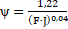,                                                             (16)

where F is the water collection area; J – water course slope.

On the basis of the described method, using formula (5), the norms of modules of maximum discharge (qm) were calculated for 21 watercourses located in various hydrological regions of the sub-mountains of Uzbekistan. Table 1 presents the materials of comparison between the calculated and actual rates of the modules of maximum discharges for some rivers of the Chirchik-Akhangaran hydrological region of Uzbekistan.

Table 1.

Comparison of calculated and actual values ​​m odul maximum water discharge on some rivers of the Chirchik-Akhangaran hydrological region

 River - post F, км2 q m м3/с∙ км2 q2m м3/с∙ км2 Difference between calculated and actual values м3/с∙ км2 % Karankulsay - Karankul 15,6 1,60 1,16 −0,44 −27,5 Aktashsay – Aktash 19,3 0,88 0,95 +  0,07 +7,95 Parkentsai − Kirghiz 39,7 0,82 0, 69 - 0,13 -15,8 Altinbelsai - Kyrgyz 19,1 1,06 1,10 +0,04 +3,77

Note: F is the water collection area; qm - actual rate modulus of maximum flow rate; q2m - calculated module norms of maximum water flow rates according to formula (5).

Comparison between the calculated and actual values ​​of the maximum discharge rate in general for the watercourses of Uzbekistan showed good convergence, while the standard deviation was σ = 27.6%. If we take into account the accuracy of determining the actual value of the measured maximum water flow rate σ = ± 18% (according to experts), then the error in determining the maximum mudflow water flow rate according to the proposed method will be equal to: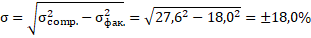Here we note that in order to calculate the maximum rainfall discharge of the required supply, its variation factors Cvm and asymmetry Csm should be determined. The variation factors of the maximum discharge for the rivers under consideration in the territory of Uzbekistan turned out to be well related to the heights of water collections:

Cv =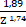R = 0.71.                                                           (17)

The third statistical parameter for calculating the availability of maxima is the asymmetry factor Csm is found according to its given ratio Ksm to the variation factor to Cvm, that is:

Кsm = Сsm / Сvm

or

Сsm = Кsm Сvm.                                                             (18)

According to studies  of the sub-mountain rivers of Uzbekistan, the value of Кsm varies from 1.50 to 3.77.

Conclusions

As a result of the research conducted by the author

• formulated the basic requirements for the design of small bridge crossings of foothill mudflow channels, as well as the principles of hydrological calculations when determining their holes;
• a method for calculating the openings of bridge crossings, based on the use of hydromorphological dependencies, does not require additional field work;

References:

1. Andreev O.V. Design of bridges. Moscow: Avtotransizdat. −1960. −292 p.

2. Begam L.G., Volchenkov G.Ya. Water-carrying capacity of bridges and pipes.-M .: Transport, 1973.-192 p.

3. Boldakov E.V. Watercourse crossings. Moscow: Transport. − 1965. − 422 p.

4. Bolshakov VA, Kurganovich AA. Calculations of the runoff and openings of small bridges and dorzhnykh pipes. Kiev. From-de "BudIvelnik" .- 1966.-148 p.

5. Vafin R.G. Floods and mudflows in Uzbekistan. Reference manual. MCHJ "O'zsuvloyiha". Tashkent. − 2012. − 138 p.

6. Vinogradov M.I. Approximate determination of the type of flow in the hole of small road culverts. // Proceedings of TsNIIS, issue 242, 1967.-pp. 54-58.

7. Denisov V.M. On the calculation of the maximum water discharge of rainfall floods // Tr. SANIIGMI. 1986. - no. 119 .-- p. 23 - 43.

8. Denisov Yu.M. The general structure of the formula for calculating the maximum discharge of mudflow floods // Proceedings of NIGMI.-2007.-Issue 4 (249) .- p. 3-14.

9. Dergacheva I.V. Using an information and diagnostic system to assess the risk of flash floods in Uzbekistan. // zbekiston geography of zhamiyati akhboroti. Tashkent, 2019. − 56 − zild − 187 −192 bet.

10. Dergachva I.V. Flash flood caused by the breakthrough of high − mountain lakestjhe on their of Uzbekistan \\ European seience rview. No. 9-10, 2018.-P.75-78 /

11. Myagkov S.V., Dergacheva I.V., Myagkov S.S. Mudflows in Central Asia // Sel toshқinlari va kўchki ҳodisalari. Toshkent Ministry of Emergency Situations − 2109. − 78−82 b.

12. Nevsky V.V. Use of hydromorphological relationships to determine the maximum flow rates of rivers. \\ Transport construction. 1971, No. 11, pp. 41−42.

13. Perevoznikov BF Calculation of the maximum flow in the design of road structures. Moscow: Transport, 1975.– 304 p.

14. Rothenburg I.S., Volnov V.S., Polyakov M.P. Bridge crossings. M .: Higher school, 1977. − 328 p.

15. Salimova B.D. Method for calculating the maximum discharge of rainwater from small catchments.-T.: - 2011.- 96 p.

16. Tulaganov A.X., Tulaganov S.X. Flood hydrology and protection of roads from floods. Tashkent .: "Economy-Finance". −2014. - 94 pages.

17. Tulyaganov A.X, Salimova B.D. Calculation of water and flood characteristics in the design of drainage structures on highways. Tashkent, “Economy − Finance”, 2016. − 156 pages.

18. Tulyaganov A.X. The problem of calculating the maximum consumption of rainwater in the basins of small mountain rivers // Information of the Geographical Society of Uzbekistan. Tashkent, 2019. − 56 − vol. − 250 −254 pages.

19. Tulyaganov A.Kh., Makhkamov B.R., Mamedova K.Z. The value and calculation of the selenium content coefficient in the foothill small rivers of the Fergana Valley // Universum: Technical Sciences: electronic scientific journal. 2020.No. 6 (75). - pp. 2-5.

20. Tulyaganov A.Kh., Makhkamov B.R. On the issue of determining the level of high water in the wake of floods on the supports of existing bridges (on the example of small foothill rivers in Uzbekistan). // Univyrsum: technical sciences. Archive of issues of the journal of technical sciences. 2020, No. 9 (78). −5−8 s.

21. Fedotov G.A. Research and design of bridge crossings. −M .: Publishing center “Academy”, 2005. − 304 p.

22. Fleishman S.M. They sat down. - Publishing house 2-in, revised, and add. - L.: Gidrometeoizdat, 1978. –310 p.

Информация об авторахPhD in Engineering, Associate Professor, Exploration and automobile road designing department, Tashkent State Transport University, Republic of Uzbekistan, Tashkent

канд. техн.наук, доцент, кафедра изысканий и проектирования автомобильных дорог, Ташкентский Государственный Транспортный университет, Республика Узбекистан, г. Ташкент